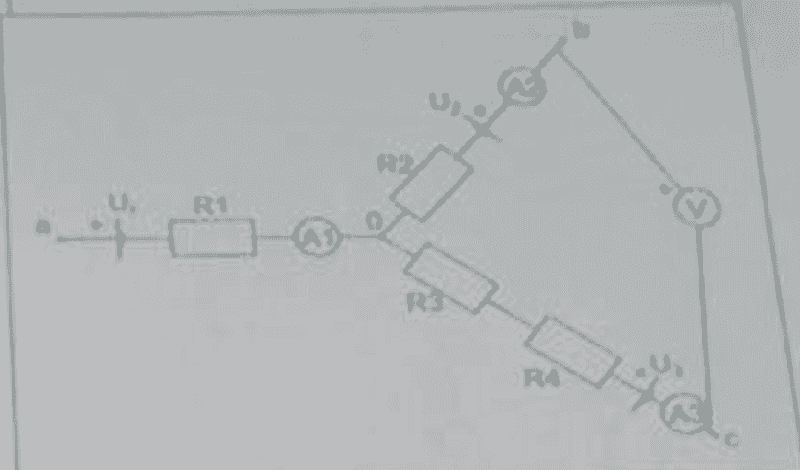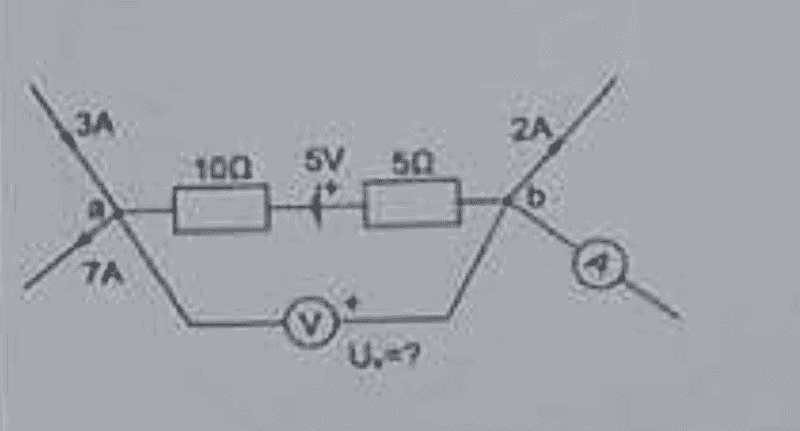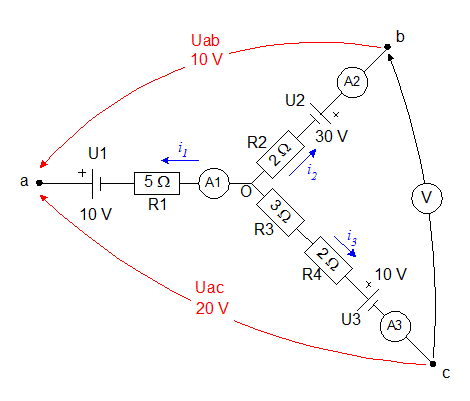# Voltmeter and ammeter calculation in a circuit

• Engineering

## Homework Statement

[/B]
Hello, basically I have some tasks about calculating the reading of voltmeter and ammeter in a circuit, I can solve these with simpler circuits, where voltmeter is reading a few elements, but as in the ones below, I'm not sure where to begin and what to add into the calculations

In this example, would I have to calculate the total voltage of all elements and the current? as in:
Rtotal = R1 + R2 + R3 + R4
Utotal (Voltage) = U1 + U2 + U3
in order to get I = Vtotal/Rtotal which would then help with the voltmeter calculation using U = I * R?

and then what would the step after that be to get the voltmeter reading calculated?Similarly here, except here we also have to apply kirchhoff law, from which side should I begin to calculate and why?## Homework Equations

Rtotal = R1 + R2 + R3 + R4
Utotal = U1 + U2 + U3
I = V/R
U[voltmeter] = I * R

## The Attempt at a Solution

So I tried what I said above, calculating the total resistence, voltage and current but from there on I don't know what to use to get the voltmeter reading, which elements are relevant for it and if I include them all or only on certain branches (branches B and C in first image)

In second one, I would know how to calculate the reading of voltmeter through ohm law formulas, but I don't know which side to start off using kirchhoff law, I know that all current that enters must leave, so in the image 7A is leaving, 3A is entering, 2A is entering on the right side, that means 2A is coming from I assume where ammeter is?

If anyone could help me with these it'd be appreciated

#### Attachments

Last edited:

Khashishi
For the first one, is there anything attached to the points a, b, c? If not, then there isn't a completed circuit, and no current will flow.

The second one is simple. What do you know about a voltmeter? Ideally, how much current flows through a voltmeter? You have the current coming out one end, so how much current is coming in the other end?

anorlunda
Staff Emeritus
This sounds like homework, so I moved it to a homework forum. In the future, please post in the appropriate forum and please fill out the templates in the homework forums.

One more thing is required. You must should your attempt at solving the problem yourself. Show us your work. We are not allowed to do the work for you and just give you the answer.

For the first one, is there anything attached to the points a, b, c? If not, then there isn't a completed circuit, and no current will flow.

The second one is simple. What do you know about a voltmeter? Ideally, how much current flows through a voltmeter? You have the current coming out one end, so how much current is coming in the other end?

1st:

It's just a part of some circuit and I just have to calculate reading of voltmeter using what's given in it, which is:
R1 = 5 Ohms
R2 = 2 Ohms
R3 = 3 Ohms
R4 = 2 Ohms
U1 = 10V
U2 = 30V
U3 = 10V
Uac = 20V
Uab = 10V

So with that I need to determine what would the voltmeter and the ammeter say at their positions

2nd:

Well voltmeter has high resistance which means current won't flow through it, so if I have 7A leaving, and 3A + 2A coming in, I'd assume 2A more is coming from where ammeter is?

This sounds like homework, so I moved it to a homework forum. In the future, please post in the appropriate forum and please fill out the templates in the homework forums.

One more thing is required. You must should your attempt at solving the problem yourself. Show us your work. We are not allowed to do the work for you and just give you the answer.

Thank you, I apologize for wrong post and I've updated the main post with the template

Khashishi
It's actually very hard to tell which direction the arrows are pointing on the currents in the picture.

7A is leaving the others are coming in

Edit: I don't even need an exact answer I just need to someone to tell me the concept of solving it in the cases as above, basically steps

gneill
Mentor
Hi matthewc,

In both cases you should be able to apply KCL and KVL (and maybe a dash of Ohm's law) to write equations that will allow you to solve for the voltages and currents. Take your first example:I've added the information that you provided about the part values and known potential differences, as well as some assumed current directions for the branches. You should be able to write two KVL equations for the pair of "loops" where Uab and Uac complete them, and of course use KCL at node O for a third equation relating the currents. Solve for the currents. As for the voltmeter reading, you should be able to find that by inspection now that the diagram has been labelled